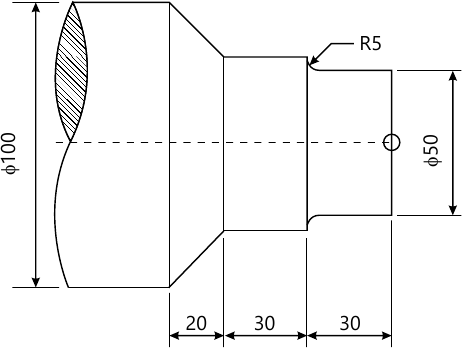MU Mechanical Engineering (Semester 7)
December 2015
Total marks: --
Total time: --
INSTRUCTIONS
(1) Assume appropriate data and state your reasons
(2) Marks are given to the right of every question
(3) Draw neat diagrams wherever necessary

1 (a) Explain the concept of homogenous coordinate system and its significance.
5 M
1 (b) Explain the difference in adaptive and feedback control & in what circumstances the Adaptive Control is preferred?
5 M
1 (c) What are the major steps to solve the problem using FEM? Whether it gives exact answer? Why it has become popular?
5 M
1 (d) Explain Drive-part check (DPC) surface syntax in APT programming by considering a suitable example.
5 M

2 (a) Write a Manual part program for finishing the following forged component as shown in Fig. 1. Illustrate the meaning of each code used in the program and the tool movement by showing the tool path.
Take spindle speed as 1000 rpm and feed rate 0.5 mm/rev. use the diametral format for programming.8 M
2 (b) Write the program for the above component (fig. 1) assuming the raw billet size of dia. 100 mm and length 150 mm, using the canned cycle for rough turning followed by finished turning, keeping the finishing allowance as 0.5 mm and 0.3 mm along Z and X axis respectively.
6 M
2 (c) Find the Transformation that rotates the objects points through 30 degree about point (1, 2). To what does the point (2, 3) maps?
6 M

3 (a) List the different types of hidden line/surface (HLR/HSR) removal algorithms explain any one in detail.
10 M
3 (b) Explain Selective Leaser Sintering (SLS) and how is it different from 3D printing?
10 M

4 (a) Explain the elements of computer integrated manufacturing and their functioning.
8 M
4 (b) what do you mean by parametric & nonparametric expression of curves? What are the advantages of parametric curves? Express the equation of Line & circle in the parametric form.
8 M
4 (c) What are the different types of errors which may get introduced while converting the CAD solid model into RPT compatible format?
4 M

5 (a) Find the Transformation matrix which aligns a given vector V=aI+oJ+cK in three dimensional space with positive Z axis.
10 M
Explain:
5 (b) (i) P & H refinement methods of CAE.
5 M
5 (b) (ii) Compare Bezier and B spline blending functions.
5 M

Explain any four of the following (any four):
6 (a) Automated guided Vehicle.
5 M
6 (b) Rapid Tooling
5 M
6 (c) AI in Design
5 M
6 (d) Applications of RPT.
5 M
6 (e) Role of CAD/CAM in CIM.
5 M

More question papers from CAD / CAM / CIM